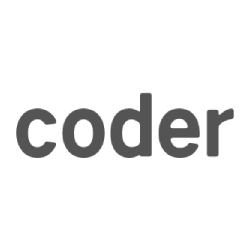# scala (3) Function 和 Method

scala 定义函数的关键字是 val

``````object FunctionDemo {

//通用的定义格式
val f1 = (x: Int, y: Int) => {
x + y
}

//先定义函数的参数列表类型，具体的函数参数在函数体中定义
val f2: (Int, Int, Int) => Int = {
(x, y, z) => {
x + y + z
}
}

def main(args: Array[String]): Unit = {
val v1 = f1(1, 2)
println(v1)

val v2 = f2(1, 2, 4)
println(v2)

}

}      ``````

//方法的返回值不需要使用return关键字，同时方法的最后一条语句的返回值作为整个方法的返回值

//[注意]：如果一个方法有返回值，那么方法的最后一条语句的返回值，一定要和方法的返回值类型保存一致

def m1(x: Int, y: Int): Int = {

var a = 1

a += 2

x + y

}

``````//可以省略掉方法的返回值类型,scala会自动根据最后一条语句的返回值推断出方法的返回值类型
def m2(x: Int, y: Int) = {
x + y
}      ``````
``````//如果方法没有返回值，可以使用Unit来标注,变现为“()”，类似于java中的void
def m3(x: Int, y: Int): Unit = {
x + y
}      ``````
``````//也是没有返回值的方法，在参数列表括号后面直接添加方法体{}，我们称这种方法为过程
def m3_3(x: Int, y: Int) {
println(x + y)
}      ``````
``````//先定义方法参数列表类型，具体的参数名称在方法体中
def m4: (Int, Int, Int) => Int = {
(x, y, z) => {
x + y + z
}
}      ``````
``````//柯理化
def m5(x: Int)(y: Int) = {
x + y
}

//柯理化
def m6(x: Int) = (y: Int) => {
x + y
}      ``````
``````//如果定义一个方法，方法名称后面的参数列表为空，那么在调用的时候可以加括号，也可以不叫括号
def m7() = {
println("hello world")
}

//如果定义一个方法，方法没有参数列表，那么在调用的时候也不能加“()”,否则编译不通过
def m8 = {
println("hello world")
}      ``````
``````//递归方法要求我们必须写明方法的返回值类型，不能省略掉否则报错
def m9(num: Int): Int = {
if (num <= 0) 0 else m9(num - 1)
}

//当参数个数不固定时，那么这时候可以将参数定义为可变参数，可变参数要求是方法的最后一个参数
def m10(name: String, nums: Int*): Unit = {
var sum = 0
for (num <- nums) {
sum += num
}
println(name + "=" + sum)
}      ``````
``````//在Scala中，有时我们调用某些方法时，不希望给出参数的具体值，而希望使用参数自身默认的值，
// 此时就定义方法时使用默认参数
//在调用方法的时候，赋值是从左往右依次赋值，所以说需要把没有默认值的放在最前面
def m11(age: Int, name: String = "旺财", sex: String = "男") = {
println(name + "=" + age + "=" + sex)
}      ``````
``````def main(args: Array[String]): Unit = {
/*   val sum = m1(1, 2)
println(sum)
*/
/*    val sum = m2(1, 3)
println(sum)*/

// println(m3(1, 2))

//println(m5(2)(3))
//    println(m6(3)(4))
//m7
// m8
// m3_3(1, 3)
// println(m9(9))
// m10("旺财", 1, 2, 3, 4, 5)

// m11(12)
//带参数名调用,传入参数就可以无序
m11(sex = "公", age = 12,name = "zs")

}

}      ``````

``````object MethodToFunctionDemo {

def m1(x: Int, y: Int) = {
x + y
}
/**
* 接收三个参数
*
* @param f 它是一个函数，接收两个参数，返回值是Int类型，在传入这个参数的时，传入进来的参数必须符合函数的签名
* @param y 普通参数
* @param x 普通参数
* @return
*/

def m2(f: (Int, Int) => Int, y: Int, x: Int) = {
f(x, y)
}
def main(args: Array[String]): Unit = {
//通过下划线将方法转换成函数
// val f1 = m1 _
// println(f1)

//scala会自动进行转换
//val v2 = m2(m1, 1, 2)
// println(v2)

val arr = Array(1, 2, 3, 4, 5)

val foreachFunchion = (x: Int) => {
println(x)
}

// arr.foreach(foreachFunchion)

//arr.foreach((x: Int) => {println(x) })

//arr.foreach((x: Int) => println(x))
// arr.foreach((x) => println(x))
// arr.foreach(println(_))

val filterFunction = (x: Int) => {
x > 3
}
arr.filter(filterFunction).foreach((x:Int)=>println(x))
}
}      ``````

08-07

09-27

08-07

08-07

08-07

08-07

08-07

08-07

08-07

08-07

08-07

08-07

08-07

08-07

08-07

08-07